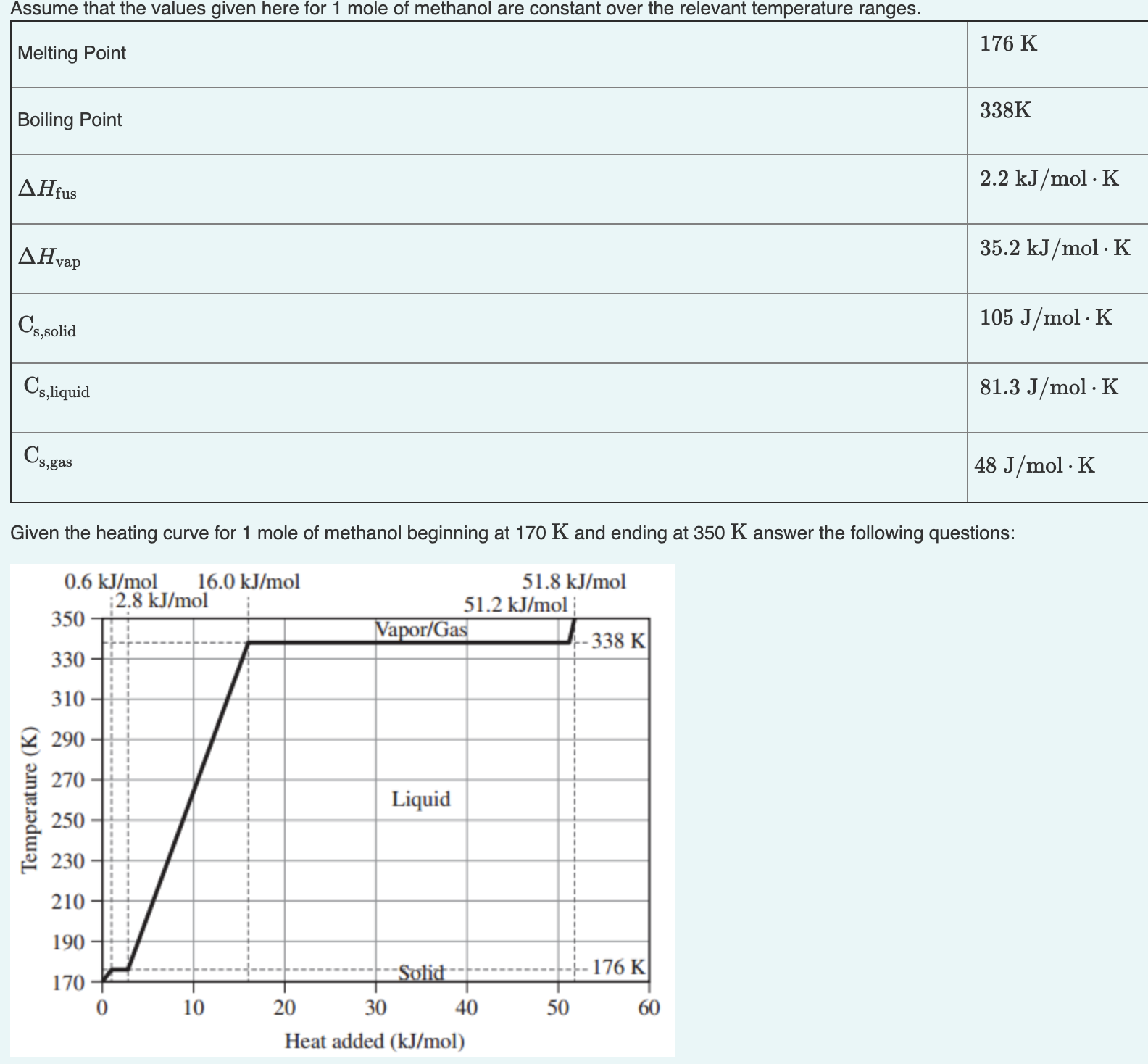# Assume that the values given here for 1 mole of methanol are constant over the relevant temperature ranges. Given the heating curve for 1 mole of methanol beginning at 170 K and ending at 350 K answer the following questions: A.) What is the value of qsolid for methanol? Express your answer to two significant figures. B.)What is the value of qliquid for methanol? C.)What is the value of qgas for methanol? Express your answer to two significant figures.